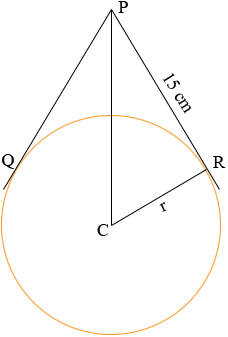SEARCH HOMEMath Central Quandaries & QueriesQuestion from rogerson, a student: The length of the tangent to a circle is 15 cm. If the angle between the two tangent lines to the circle is 28 degrees, what is the radius of the circle?Rogerson,The measure of the angle RPQ is 28o and thus the measure of the angle RPC is 14o. Since CR is a radius of the circle and PR is tangent to the circle, angle CRP is a right angle. What trig function relates the angle RPC and the sides CR and RP?

PennyMath Central is supported by the University of Regina and The Pacific Institute for the Mathematical Sciences.